### Home > GC > Chapter 12 > Lesson 12.1.2 > Problem12-18

12-18.
1. Use the relationships in each diagram below to solve for the given variables.

1.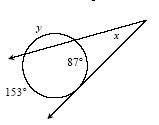Homework Help ✎

2.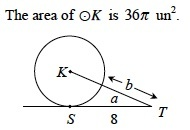3.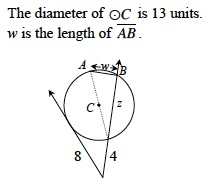Homework Help ✎

4.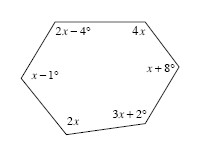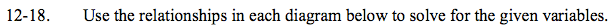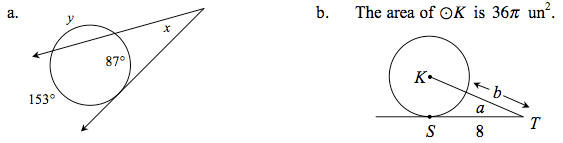The secant-tangent angle is half the difference between the major and minor arcs.

The total measure of all the arcs in a circle is 360°.

$x=\frac{153^{\circ}-87^{\circ}}{2}=33^{\circ}$

The area of a circle is πr2.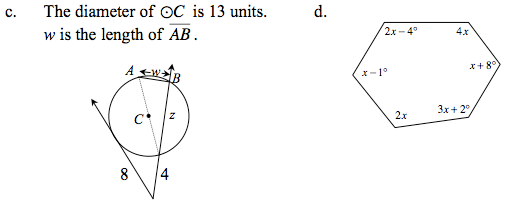Refer to Lesson 11.2.3 for help with the relationships.